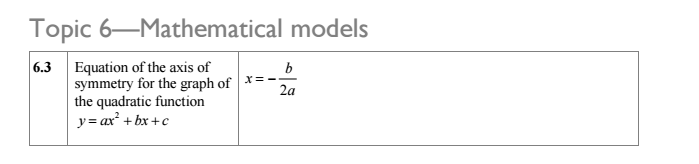### 03. Functions

 Relevant textbook pages 4.1 Functions p134-146    - Domain and range of a function    - Graph of a function    - Function notation    - Functions as mathematical models4.2 Linear models p147-152    - Linear models involving simultaneous equations4.3 Quadratic models p152-165    - Sketching quadratic graphs    - Intersection of two functions    - Finding the equation of a quadratic function from its graph    - Quadratic models4.4 Exponential models p166-174    - Exponential functions and their graphs    - Graphs of exponential functions    - Applications of exponential functions4.5 Graphs of functions in the form ... p175-187    - Cubic functions    - Graphs of functions when the power of x is a negative integer    - Graphs of more complex functions    - Sketching more complex graphs  4.6 Using a GDC to solve equations p187-1884.7 Graphs of real life situations p188-192Review exercises p192-197Paper 1 style questionsPaper 2 style questionsEnd of chapter summary p198-199 Relevant sections from the IBO Exam Formula BookletTexas Instruments Instructions for Solving Simultaneous Equations on the GDC